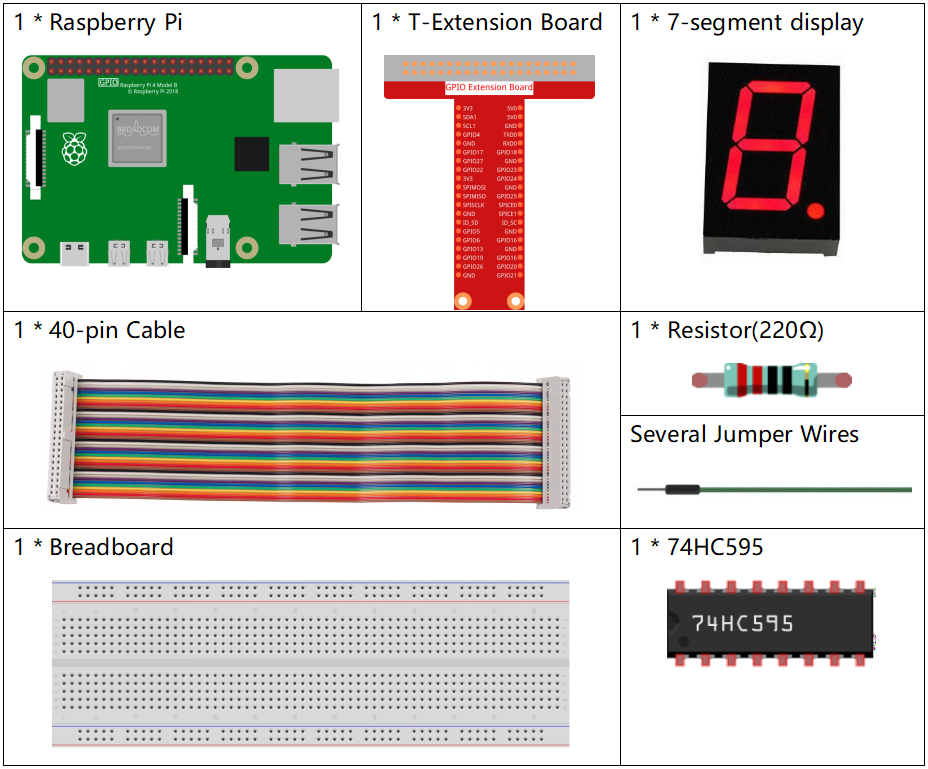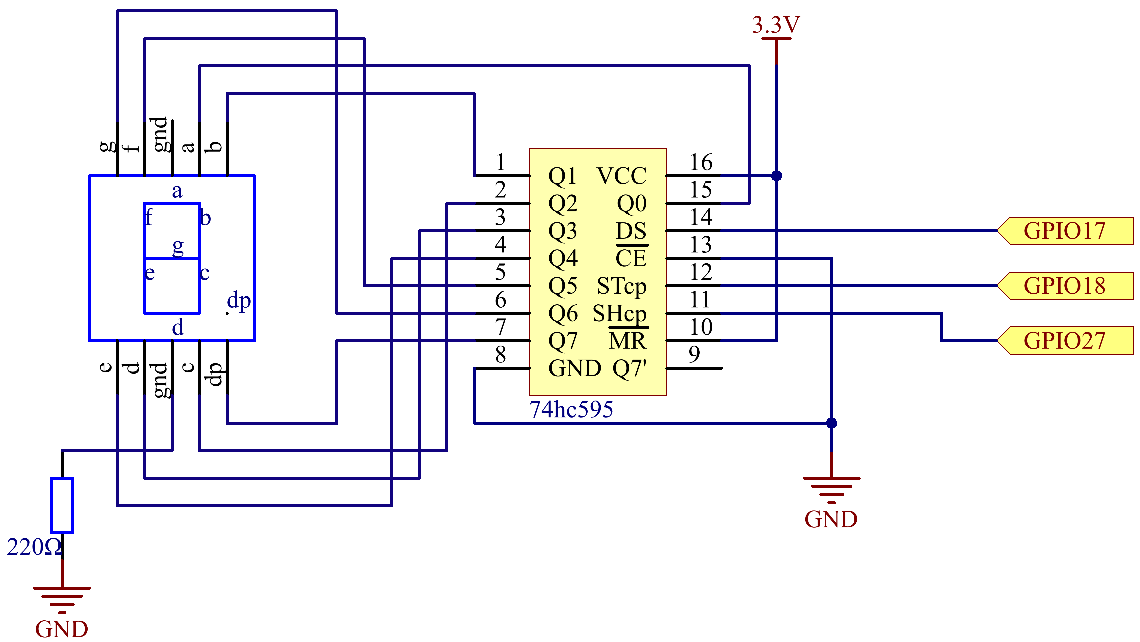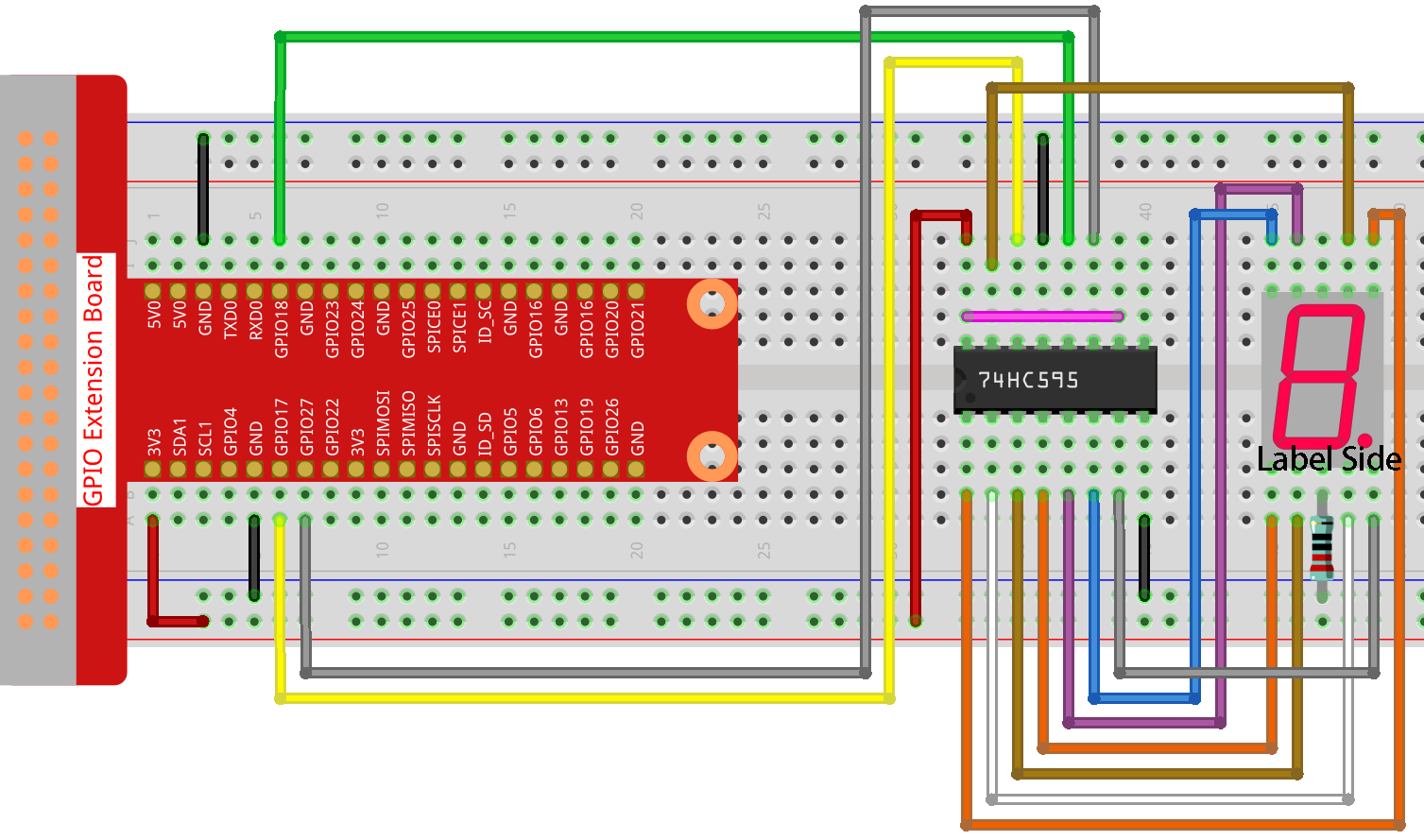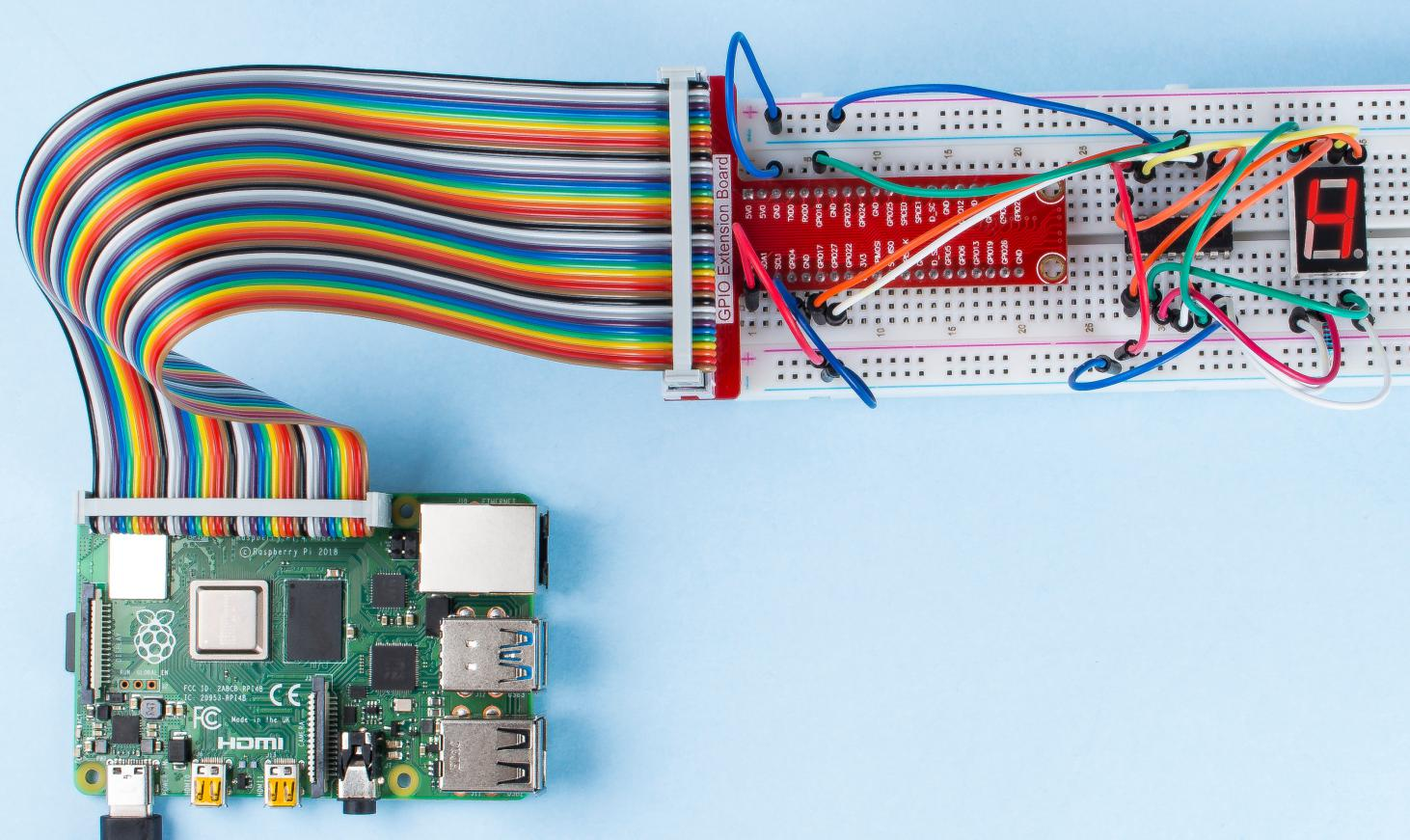# 1.1.4 7-segment Display¶

## Introduction¶

Let’s try to drive a 7-segment display to show a figure from 0 to 9 and A to F.

## Components¶## Schematic Diagram¶

Connect pin ST_CP of 74HC595 to Raspberry Pi GPIO18, SH_CP to GPIO27, DS to GPIO17, parallel output ports to 8 segments of the LED segment display. Input data in DS pin to shift register when SH_CP (the clock input of the shift register) is at the rising edge, and to the memory register when ST_CP (the clock input of the memory) is at the rising edge. Then you can control the states of SH_CP and ST_CP via the Raspberry Pi GPIOs to transform serial data input into parallel data output so as to save Raspberry Pi GPIOs and drive the display.## Experimental Procedures¶

Step 1: Build the circuit.Step 2: Go to the folder of the code.

```cd /home/pi/raphael-kit/nodejs/
```

Step 3: Run the code.

```sudo node 7-segment_display.js
```

After the code runs, you’ll see the 7-segment display display 0-9, A-F.

Code

```const Gpio = require('pigpio').Gpio;

const segCode = [0x3f, 0x06, 0x5b, 0x4f, 0x66, 0x6d, 0x7d, 0x07, 0x7f, 0x6f, 0x77, 0x7c, 0x39, 0x5e, 0x79, 0x71];

const SDI = new Gpio(17, { mode: Gpio.OUTPUT });
const RCLK = new Gpio(18, { mode: Gpio.OUTPUT });
const SRCLK = new Gpio(27, { mode: Gpio.OUTPUT });

function hc595_shift(dat) {
for (let j = 0; j < 8; j++) {
let code = 0x80 & (dat << j);
if (code != 0) {
code = 1;
}
SDI.digitalWrite(code);
SRCLK.trigger(1,1);
}
RCLK.trigger(1,1);
}

let index = -1;
setInterval(() => {
index = (index+1)%16;
hc595_shift(segCode[index]);
}, 1000);
```

Code Explanation

```const segCode = [0x3f,0x06,0x5b,0x4f,0x66,0x6d,0x7d,0x07,0x7f,0x6f,0x77,0x7c,0x39,0x5e,0x79,0x71];
```

Define a hexadecimal (common cathode) segment code array from 0 to F.

```const SDI = new Gpio(17, { mode: Gpio.OUTPUT });
const RCLK = new Gpio(18, { mode: Gpio.OUTPUT });
const SRCLK = new Gpio(27, { mode: Gpio.OUTPUT });
```

Initialize pins 17, 18, and 27 as output mode, and copy them to `SDI`, `RCLK`, and `SRCLK` respectively.

```function hc595_shift(dat) {
for (let j = 0; j < 8; j++) {
let code = 0x80 & (dat << j);
if (code != 0) {
code = 1;
}
SDI.digitalWrite(code);
SRCLK.trigger(1,1);
}
RCLK.trigger(1,1);
}
```

Implement a `hc595_shift` function to convert the fields in the array `segCode` into numbers and display them on the digital tube.

```let code = 0x80 & (dat << j);
if (code != 0) {
code = 1;
}
SDI.digitalWrite(code);
```

Assign the dat data to SDI(DS) by bits. Here we assume dat=0x3f(0011 1111, when j=2, 0x3f will shift right(<<) 2 bits. 1111 1100 (0x3f << 2) & 1000 0000 (0x80) = 1000 0000, is true. At this time, 1 is written to SDI.

```SRCLK.trigger(1,1);
```

Generate a rising edge pulse and move the DS data to the shift register.

`trigger(pulseLen, level)`
• pulseLen - pulse length in microseconds (1 - 100)

• level - 0 or 1

Sends a trigger pulse to the GPIO. The GPIO is set to level for pulseLen microseconds and then reset to not level.

```RCLK.trigger(1,1);
```

Generate a rising edge pulse and move the data from the shift register to the storage register.

```let index = -1;
setInterval(() => {
index = (index+1)%16;
hc595_shift(segCode[index]);
}, 1000);
```

Finally, use the function `hc595_shift()` to convert the fields in `segCode` and display them through the digital tube.

## Phenomenon Picture¶